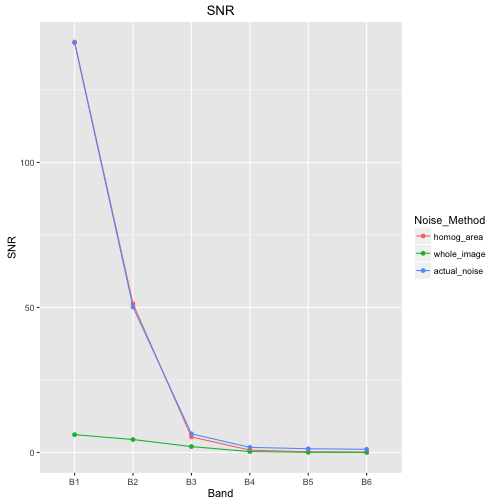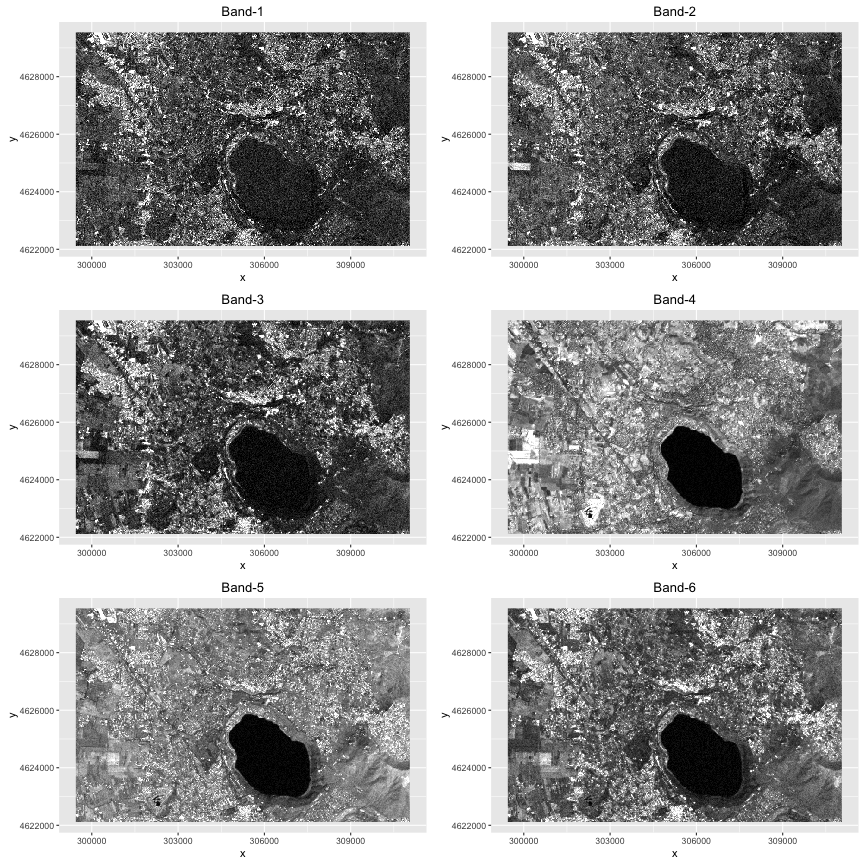# The effect of noise estimation in MNF denoising

``````knitr::opts_chunk\$set(fig.path = "figure/CompareOrig2invMNF_", warning=FALSE)

require(rgdal)
require(raster)
require(ggplot2)
require(reshape2)
#require(gstat)
require(usdm)
require(gridExtra)
require(RStoolbox)

#resdir <- "/media/alobo/LACIE500/MNFtests/results/SPy"
resdir <- "/Volumes/LACIE500/MNFtests/results/SPy"
``````

The Minimum Noise Fraction (MNF ) transform of a multi- or, more typically, hyper-spectral image results on a new multi-band image in which new “bands” are ordered in terms of increasing noise. This is achieved in a similar way as PCA, but the eigenanalysis in MNF is performed considering both the covariance matrix of the image and the covariance matrix of the noise.
A common use of the MNF trasform is discarding those components with low SNR (actually these components are set to the mean value of the component) and then applying the inverse transform in order to obtain an image as the original one but with reduced noise. MNF relies on an estimate of the noise statisitcs and this is actually a delicate step. The best is disposing of a set of “dark images” (images acquired with no input signal) in which noise statistics can be measured. In case this is not possible, noise statistics may be derived from an homogeneous area in the image. Not using a correct estimate of the noise (for example, estimating noise over a non-uniform area) has a very negative impact on the quality of the denoised image. By adding a known noise to a multi-spectral image and denoising back, we compare the effects of a noise estimated over a homogeneous area and the extreme case of using the entire image for the noise estimation.
 Green et al 1988. “A Transformation for Ordering Multispectral Data in Terms of Image Quality with Implications for Noise Removal.” Geoscience and Remote Sensing, IEEE Transactions on 26 (1): 65–74

## 1. Data input

Original image is a subscene that I made from of a Landsat image available from
https://dl.dropboxusercontent.com/u/3180464/MNFimas.zip

Modify paths as needed

### 1.1 Original image

``````ori <- brick(file.path(datadir,"mini.img"))
names(ori) <- paste0("B",1:nlayers(ori))
``````

### 1.2 Noised image

``````set.seed(1834)
z <- ori
z[] <- rnorm(length(ori),sd=1)
orin <- ori+z/100
rm(z)
#My actual images are
names(orin) <- names(ori) <- paste0("B",1:6)
``````

### 1.3 MNF and MNF-denoised images

These images were created by

• Estimate noise from noised image “orin”
• For invpy1, noise was estimated over a uniform area in orin
• For invpy2, all the image orin was used to estimate the noise
• Calculate MNF transform
• Keep only components with SNR over a certain threshold
• Inverse transform

MNF denoising was performed using PySpy by Thomas Boggs
and based on the example provided in algorithms.py

Read PySpy results: MNF and MNF-denoised images:

``````mnfima <- brick(file.path(resdir,"mininoiseMNFSPy.img"))
mnf2ima <- brick(file.path(resdir,"mininoiseMNFSPy2.img"))
invpy1 <- brick(file.path(resdir,"minidenoiseSPy.img"))
invpy2 <- brick(file.path(resdir,"minidenoiseSPy2.img"))
invpy3 <- brick(file.path(resdir,"minidenoiseSPy3.img"))
names(invpy1) <- names(invpy2) <- names(invpy3) <- names(ori)
``````

``````snrmat <- read.csv(file.path(resdir,"snrmat.csv"),header=FALSE)
names(snrmat) <- c("homog_area","whole_image","actual_noise")
snrmat\$Band <- paste0("B",1:6)
msnrmat <- melt(snrmat,id.vars="Band",variable.name = "Noise_Method")
names(msnrmat) <- "SNR"
ggplot(data=msnrmat) +
geom_point(aes(x=Band, y=SNR,color=Noise_Method)) +
geom_line (aes(x=Band, y=SNR,group=Noise_Method,color=Noise_Method)) +
ggtitle("SNR")
``````With an SNR threshold of 5, we have discarded (set to their respective mean value) the last 3 MNF components for the denoising in all cases.

## 2. Display images

### 2.1 Noised image

``````ggn1 <- ggR(orin[],stretch="lin") + ggtitle("Band-1")
ggn2 <- ggR(orin[],stretch="lin") + ggtitle("Band-2")
ggn3 <- ggR(orin[],stretch="lin") + ggtitle("Band-3")
ggn4 <- ggR(orin[],stretch="lin") + ggtitle("Band-4")
ggn5 <- ggR(orin[],stretch="lin") + ggtitle("Band-5")
ggn6 <- ggR(orin[],stretch="lin") + ggtitle("Band-6")
grid.arrange(ggn1,ggn2,ggn3,ggn4,ggn5,ggn6, ncol=2)
rm(ggn1,ggn2,ggn3,ggn4,ggn5,ggn6)
``````### 2.2 MNF transform (noise estimated over homogenous area)

``````ggm1 <- ggR(mnfima[],stretch="lin") + ggtitle("MNF-1")
ggm2 <- ggR(mnfima[],stretch="lin") + ggtitle("MNF-2")
ggm3 <- ggR(mnfima[],stretch="lin") + ggtitle("MNF-3")
ggm4 <- ggR(mnfima[],stretch="lin") + ggtitle("MNF-4")
ggm5 <- ggR(mnfima[],stretch="lin") + ggtitle("MNF-5")
ggm6 <- ggR(mnfima[],stretch="lin") + ggtitle("MNF-6")
grid.arrange(ggm1,ggm2,ggm3,ggm4,ggm5,ggm6, ncol=2)
rm(ggm1,ggm2,ggm3,ggm4,ggm5,ggm6)
``````